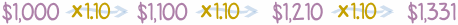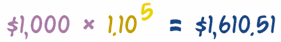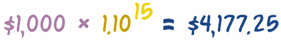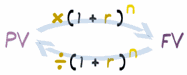# 导出复利公式## 做个公式

￥1,000.00 +（￥1,000.00 × 10%）= ￥1,100.00（注意：把利率除以 100 来转换为小数：10% = 10/100 = 0.10，去这里学习百分比。）

### 公式在任何年份都适用：

• 我们可以这样求下一年的利息：￥1,100 × 1.10 = ￥1,210
• 再下一年：￥1,210 × 1.10 = ￥1,331
• 等等……### 这样乘 5次，我们就可以直接计算第五年的金额：

￥1,000 × 1.10 × 1.10 × 1.10 × 1.10 × 1.10 = ￥1,610.51## 公式（和上面的计算比较一下：PV = ￥1,000, r = 0.10, n = 5, and FV = ￥1,610.51）

• 如果是年利率，n 便是年数
• 如果是月利率，n 便是月数
• 等等

### 例子

…… 如果年期是 15年呢？……改变 "n" 的值：……如果年期是 5年，但利率是 6% 又怎么样？（注意乘数是 1.06，不是 1.6）

## 四个公式

FV = PV (1+r)n

• FV = 终值、
• PV = 现值、
• r = 利率（以小数表达）、
• n = 期数

 FV = PV (1+r)n 求终值e，如果已知现值、利率和期数。 PV = FV / (1+r)n 求现值，如果已知终值、利率和期数。 r = ( FV / PV )1/n - 1 求利率，如果已知现值、终值和期数。 n = ln(FV / PV) ln(1 + r) 求期数，如果已知现值、终值和利率

## 求现值

### 例子：小山想在 5年后有 ￥2,000，年利率是 10%，他一开始需要多少钱？

 开始： FV = PV (1+r)n 换边： PV (1+r)n = FV 每边除以 (1+r)n: PV = FV (1+r)n

### 例子（续）：

PV = ￥2,000 / (1+0.10)5 = ￥2,000 / 1.61051 = ￥1,241.84### 再举个例：你现在需要投资多少钱，才能以 8%年利率在 10年后得到 ￥10,000？

PV = ￥10,000 / (1+0.08)10 = ￥10,000 / 2.1589 = ￥4,631.93

## 求利率

### 例子：小山有 ￥1,000，他想在 5年后增长到 ￥2,000，需要的利率是多少？

 开始： FV = PV (1+r)n 换边： PV (1+r)n = FV 每边除以 PV： (1+r)n = FV PV 每边取n 次方根： 1+r = ( FV PV )1/n 每边减 1： r = ( FV PV )1/n − 1

（注意：想了解 "取 n 次方根"，去这里阅读分数指数

r = ( FV / PV )1/n − 1

### 例子（续）：

r =（￥2,000 / ￥1,000）1/5 − 1
=（2）0.2 − 1
= 1.1487 − 1
= 0.1487

0.1487 以百分比来表达是 14.87%

### 再举个例：在 20年后把 ￥1,000 变成 ￥5,000 的利率是多少？?

r =（￥5,000 / ￥1,000）)1/20 − 1 =（5）0.05 − 1 = 1.0838 − 1 = 0.0838

0.0838 就是 8.38%。所以 8.38% 的利率可以在 20年后把 \$1,000 变成 ￥5,000。

## 求期数

### 例子：小山投资的年利率是 10%，他需要多少年才能得到 ￥2,000？

 开始： FV = PV (1+r)n 换边： PV (1+r)n = FV 每边除以 PV： (1+r)n = FV / PV 用对数： ln(1+r) × n = ln( FV / PV ) 每边除以 ln(1+r)： n = ln( FV / PV ) ln(1+r)

（注意：想多了解点怎样"用对数"，去这里阅读使用指数与对数）。

"代入"数值：

### 例子（续）：

n = ln( ￥2,000 / ￥1,000 ) / ln( 1 + 0.10 ) = ln(2)/ln(1.10) = 0.69315/0.09531 = 7.27

### 再举个例：年利率是 5%，￥1,000 要几年才能变成 ￥10,000？

n = ln( ￥10,000 / ￥1,000 ) / ln( 1 + 0.05 ) = ln(10)/ln(1.05) = 2.3026/0.04879 = 47.19

47年！这不稀奇，因为金额大了十倍，而利率只有 5%。